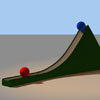#### You may also like### Lunar Leaper

Gravity on the Moon is about 1/6th that on the Earth. A pole-vaulter 2 metres tall can clear a 5 metres pole on the Earth. How high a pole could he clear on the Moon?### Which Twin Is Older?

A simplified account of special relativity and the twins paradox.### Whoosh

A ball whooshes down a slide and hits another ball which flies off the slide horizontally as a projectile. How far does it go?

# Earth Orbit

##### Age 16 to 18Challenge Level

The force which drives the motion of the planets around the sun is gravity and Newton showed that this force is inversely proportional to the square of the distance of the planet from the sun. The constant of proportionality is called Newton's constant $G$.

If the mass of the planet is $m$ and the mass of the sun is $M$ then the force between the two is $$F = -\,\frac{GmM}{r^2}\;,\quad\quad G = 6.674 \times 10^{-11}\mathrm{m}^3\mathrm{kg}^{-1}\mathrm{s}^{-2}\;.$$ In polar coordinates in the plane of motion, the equation of motion is$$\frac{d^2 r}{dt^2}-\frac{h^2}{r^3} = -\frac{GM}{r^2}$$ where the angular momentum $h$ of the planet is given by $$h = r^2\frac{d\theta }{dt}\;.$$ This equation is very tricky to solve directly, but making the substitution$$u = \frac{1}{r}$$ leads to an inhomogenous linear second order differential equation.

Find this equation and show that its solution is$$u=GM/h^2 +A\cos \theta + B \sin \theta\;.$$ Show that numbers $e$ and $f$ can be found so that$$h^2 = GMr(1+e\cos(\theta+f))\;.$$

For a fun and very much simpler practical activity, why not try the problem Making Maths: Planet paths.

Extension: Investigate the elliptic paths observed in the solar system using the real data below. Why not try to draw a scale model of the solar system.

NOTES AND BACKGROUND
Solving the problem of the motion of the planets around the sun required the invention of differentiation and integration, credited to Newton about 350 years ago. The fact that orbits follow such beautiful, pure paths shows how elegantly the universe is put together. The results are incredibly accurate and were only challenged by Einstein whose theory of General Relativity provides very small corrections to the orbits.

Essentially, this mathematics is sufficient to send space probes all the way from earth to the far reaches of the solar system with sufficient accuracy to meet up with various planets and moons along the way.

The following table comprises real astronomical data (compiled from Wikipedia) which describe the elliptical paths taken by some key objects in our solar system.

 Name Diameter relative to Earth Mass relative to Earth Orbital radius Orbital period Inclination to sun's equator Orbital Eccentricity e Rotation period (days) The Sun 109 332946 -- -- -- -- 26.38 The Moon 0.273 0.0123 -- 29.5 days -- 0.0549 -- Halleys Comet -- -- -- 73.3 162.3 0.967 -- Mercury 0.382 0.06 0.39 0.24 3.38 0.206 58.64 Venus 0.949 0.82 0.72 0.62 3.86 0.007 -243.02 Earth 1.00 1.00 1.00 1.00 7.25 0.017 1.00 Mars 0.532 0.11 1.52 1.88 5.65 0.093 1.03 Jupiter 11.209 317.8 5.20 11.86 6.09 0.048 0.41 Saturn 9.449 95.2 9.54 29.46 5.51 0.054 0.43 Uranus 4.007 14.6 19.22 84.01 6.48 0.047 -0.72 Neptune 3.883 17.2 30.06 164.8 6.43 0.009 0.67

The actual numbers for the earth are
 Diameter Mass kg Distance from sun Orbital period Rotation time 12756 km 5.9736 x 10^24 147.1-152.1 million km 365.256366 days 23 hours 56 minutes